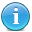# US Pet Population and Ownership Statistics Calculator

This calculator takes the most current (hourly) US population estimate from the US Census and calculates the current number of households that are pet owners based on statistical pet ownership data. It provides a further breakdown on numbers of dogs and cats.

channel->item as \$post) { //echo "
" . \$post->title; \$title = \$post->title; //get part of string \$a = \$title; \$c = explode(" ", \$a); \$b = \$c; \$d = \$c; if(\$b == "US") { \$pop = str_replace("Estimate:", "", \$d); \$pop = str_replace(",", "", \$pop); \$house = \$pop/2.64; \$s1 = 'As of ' . date("m-d-Y") . ' it is estimated that there are currently ' . number_format(\$pop) . ' people in the US, equaling ' . number_format(round(\$house)) . ' households.'; \$phouse = round(\$house * .669); \$s2 = 'Of these, ' . number_format(round(\$phouse)) . ' households own a pet.'; \$dhouse = \$house * .365; \$s3 = '' . number_format(round(\$dhouse)) . ' of US households own a dog.'; \$d1house = \$dhouse * 1.6; \$s5 = 'There is an average of 1.6 dogs per dog-owning household, totalling an estimated ' . number_format(round(\$d1house)) . ' dogs in US households. ' . number_format(round(\$d1house*.33)) . ' of owned dogs are not spayed or neutered.'; \$chouse = \$house * .304; \$s4 = '' . number_format(round(\$chouse)) . ' of US households own a cat.'; \$c1house = \$chouse * 2.1; \$s6 = 'There is an average of 2.1 cats per cat-owning household, totalling an estimated ' . number_format(round(\$c1house)) . ' cats in US households. ' . number_format(round(\$c1house*.12)) . ' of owned cats are not spayed or neutered.'; \$s7 = 'The total estimated number of dogs and cats owned in the US is ' . number_format(\$c1house + \$d1house) . ''; } } ?>Category: Pets